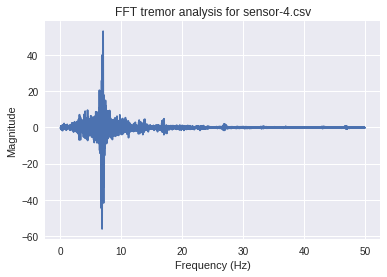# Details for `Tremor analysis notebook.ipynb`

#### Description

This notebook helps analyze tremor data via a fast fourier transform, to report the frequency and magnitude of a tremor.

# Tremor analysis notebook¶

## Acquiring data¶

This notebook expects to analyze a data file from the "Physics Toolbox Sensor Suite" phone app.

## How to use this notebook¶

• Click the "Jupyter" icon in the top left to go to your home directory.
• (You might want to "open in a new tab"!)
•  Edit the cell below with the following information:
• `target_file`: CSV data file name.
•  Run all cells from the top. There are several ways to do run cells, including...
• On your keyboard, "shift" and "enter" to run each cell (one at a time)
• Use the "Run" button above (one at a time)
• Cell → Run all to run everything at once

Run this to import libraries. They will be used by the code below.

## Clean data¶

Before doing a fourier transform, data should be a uniform sampling – there should be an equal amount of time between each data timepoint. This isn't always true in the raw data. Use an interpolation to get uniform data.

## FFT and plot¶

```/opt/conda/lib/python3.6/site-packages/numpy/core/numeric.py:531: ComplexWarning: Casting complex values to real discards the imaginary part
return array(a, dtype, copy=False, order=order)
```
Out:
`Text(0, 0.5, 'Magnitude')````Peak frequency: 7.003921174994464 Hz
Peak magnitude: 58.45948546661715
```

# Tremor analysis notebook¶

## Acquiring data¶

This notebook expects to analyze a data file from the "Physics Toolbox Sensor Suite" phone app.

## How to use this notebook¶

• Click the "Jupyter" icon in the top left to go to your home directory.
• (You might want to "open in a new tab"!)
•  Edit the cell below with the following information:
• `target_file`: CSV data file name.
•  Run all cells from the top. There are several ways to do run cells, including...
• On your keyboard, "shift" and "enter" to run each cell (one at a time)
• Use the "Run" button above (one at a time)
• Cell → Run all to run everything at once
In :
```target_file = "sensor-4.csv"

# Edit this to change the plot title. The default uses your file name.
plot_title = "FFT tremor analysis for {}".format(target_file)
```

Run this to import libraries. They will be used by the code below.

In :
```import math
import statistics

import numpy as np
import pandas as pd
import matplotlib.pyplot as plt
from scipy.fftpack import fft
from scipy.interpolate import interp1d
import seaborn
```

In :
```data_raw = pd.read_csv(target_file, parse_dates=['time'])
```

## Clean data¶

Before doing a fourier transform, data should be a uniform sampling – there should be an equal amount of time between each data timepoint. This isn't always true in the raw data. Use an interpolation to get uniform data.

In :
```# Get the median interval time for this data (i.e. typical interval)
intervals = [data_raw.index[i] - data_raw.index[i-1] for
i in range(1, len(data_raw.index))]
interval = statistics.median(intervals)

# Convert to unix epoch timestamp for easier math.
ts_raw = [t.timestamp() for t in data_raw.time]

# Set up interpolation for total gForce.
gf_raw = list(data_raw.gFTotal)
interpolate = interp1d(ts_raw, gf_raw)

# Create uniform timepoints, derive interpolated gForce values.
ts_uniform = np.linspace(ts_raw, ts_raw[-1], len(ts_raw))
avg_delta = (ts_raw[-1] - ts_raw) / len(ts_raw)
gf_uniform = interpolate(ts_uniform)
```

## FFT and plot¶

In :
```gf_fft_all = np.fft.fft(gf_uniform)
freqs_all = np.fft.fftfreq(len(ts_uniform), d=avg_delta)

target_len = int(len(freqs_all)/2)
freqs = freqs_all[1:target_len]
gf_fft = gf_fft_all[1:target_len]

plt.figure()
plt.plot(freqs, gf_fft)
plt.title(plot_title)
plt.xlabel("Frequency (Hz)")
plt.ylabel("Magnitude")
```
```/opt/conda/lib/python3.6/site-packages/numpy/core/numeric.py:531: ComplexWarning: Casting complex values to real discards the imaginary part
return array(a, dtype, copy=False, order=order)
```
Out:
`Text(0, 0.5, 'Magnitude')`In :
```# Get the maximum value, report the frequency and magintude.
peak_index = np.argmax(gf_fft)
peak_freq = freqs[peak_index]
peak_magnitude = abs(gf_fft[peak_index])

print("Peak frequency: {} Hz".format(peak_freq))
print("Peak magnitude: {}".format(peak_magnitude))
```
```Peak frequency: 7.003921174994464 Hz
Peak magnitude: 58.45948546661715
```